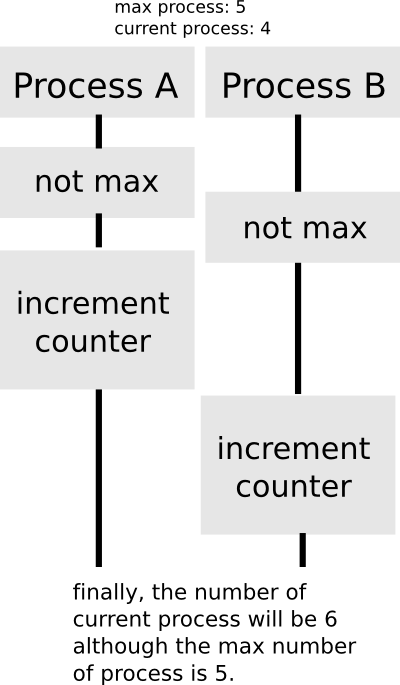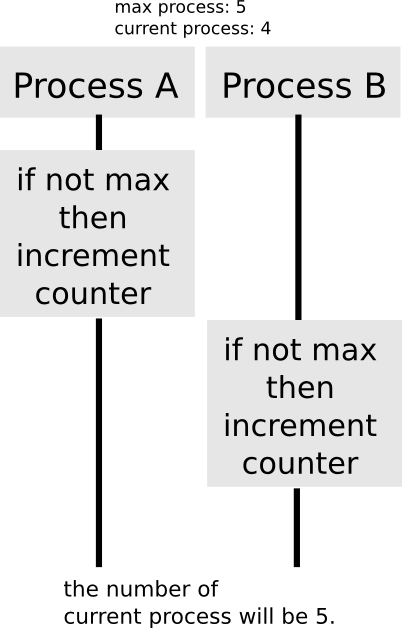## [SICP Excercise 3.47] Some observation about semaphore

7-14-2014

```(define (make-semaphore n)
(let ((the-mutex (make-mutex))
(count 0))
(define (the-semaphore m)
(cond ((eq? m 'acquire)
(the-mutex 'acquire)
(if (= count n)
(begin
(the-mutex 'release)
(the-semaphore 'acquire))
(begin
(set! count (+ count 1))
(the-mutex 'release))))
((eq? m 'release)
(the-mutex 'acquire)
(if (> count 0)
(set! count (- count 1)))
(the-mutex 'release))))
the-semaphore))
```

(I’ve Borrowed code above from Weiqun Zhang’s Blog – SICP Exercise 3.47.)

It took some time to understand the structure of semaphore. Here is the problem:

## The place of ‘acquire’

Next question that bothered me was that: does the code still work if I replace acquire method as follows?

```(define (make-semaphore n)
(let ((the-mutex (make-mutex))
(count 0))
(define (the-semaphore m)
(cond ((eq? m 'acquire)
(if (= count n)
(the-semaphore 'acquire))
(begin
(set! count (+ count 1))
(the-mutex 'release))))
((eq? m 'release)
(the-mutex 'acquire)
(if (> count 0)
(set! count (- count 1)))
(the-mutex 'release))))
the-semaphore))
```

After a little bit observation I noticed that this code has a problem:So the place of ‘acquire’ should be before (if (= count n) … :## [Scheme] Checking The Validity of The Number Passed to The List

6-1-2014

In many programming languages,
when we pass the number n to the array which the length is equal or less than n, we get an exception.

The following is the example of C:

```
int main(void){
int hoge[] = {0,1,2,3};
printf("%d", hoge); // output: 3
printf("%d", hoge); // get a warning
return 0;
}

```

We can implement this kind of exception in Scheme by using the procedure length which is introduced in SICP 2.2.1.

The code will be as follows:

```
; returns the length of a list
(define (length li)
(define (length-iter n iter-li)
(if (null? iter-li)
n
(length-iter (+ n 1) (cdr iter-li))))
(length-iter 0 li))

; check if the value passed to the list is valid
(define (cdr-chk-err li n)
(cond ((or (> n (length li)) (= n (length li)))
(error "Invalid value passed to the list. "))))

(define (list-ref n)
(lambda (x)
(cdr-chk-err x n)
(if (= n 0)
(car x)
((list-ref (- n 1)) (cdr x)))))

```

This works correctly.

```
(define l1 (list 1 2 3 4))
((list-ref 3) l1) ; returns 4
((list-ref 4) l1) ; error!

```

## [SICP 2.1.4] Some Observation about Exercise 2.16

5-31-2014

First time I saw this problem, I wondered how it works when I use fractions instead of decimals until the final operation is executed( if the number is not accurate one, then convert it to the fraction like 3.14 to 314 / 100 ).

To examine this, let’s define a function calculating some complex value.

```

(define (g n)
(define (g-iter result i)
(if (= i n)
result
(g-iter (+ (/ (/ (/ 312423 125531) (/ 2052712 7231400)) (/ 13222 113131)) result) (+ i 1)
)))
(g-iter 1 1)
)

```

The result of the operation is returned as a fraction:
(19358134129159 * 750189) * 5180721713949/19358134129159

Each term is absolutely determined value.
For getting the decimal number from this fraction value, we just need to multiply this by 1.0.

```
(* 1.0 (g 10000)) ; 750189.2676250552

```

If we use the decimal number in the calculation, the different result will show up:

```
(define (g n)
(define (g-iter result i)
(if (= i n)
result
(g-iter (+ (/ (/ (/ 312423.0 125531) (/ 2052712 7231400)) (/ 13222 113131)) result) (+ i 1)
)))
(g-iter 0 0)
)

(g 10000) ; 750189.2676250958

```

Then which number is more near the actual value?
Let’s calculate ((((312423 / 125531) / (2052712 / 7231400)) / (13222 / 113131)) * 10000) in the Wolframalpha.
Calc result

```750189.2676250551516388667249372079289168657014682584005287499...
```

It seems that the former one is more accurate.

However, the fraction-version calculation will lose its speed when the number of calculation grows up. If we try to execute function g 10000000 times, the response of the fraction-version one is cruel in contrast to the decimal-version one which outputs the result within 0.5s.

## [SICP 2.1.4] Exercise 2.11 Memo

5-30-2014

Exercise 2.11: Rewrite procedure mul-interval using Ben’s suggestion.

The default mul-interval executes 4 multiplications on every case of operations, because the process calculates p1 to p4 regardless of the signs of the endpoints.
So, the objective of this problem is to minimize the number of operations.

```
(define (mul-interval x y)
(let
((mi make-interval)
(lx (lower-bound x))
(ux (upper-bound x))
(ly (lower-bound y))
(uy (upper-bound y)))
(cond
((and (neg-int? x) (neg-int? y))
(mi (* lx ly) (* ux uy)))
((and (neg-int? x) (zero-span? y))
(mi (* lx uy) (* lx ly)))
((and (neg-int? x) (pos-int? y))
(mi (* ux uy) (* lx ly)))
((and (zero-span? x) (neg-int? y))
(mi (* ux uy) (* lx uy)))
((and (zero-span? x) (pos-int? y))
(mi (* lx uy) (* ux uy)))
((and (pos-int? x) (neg-int? y))
(mi (* ux uy) (* lx ly)))
((and (pos-int? x) (zero-span? y))
(mi (* ux ly) (* ux uy)))
((and (pos-int? x) (pos-int? y))
(mi (* lx ly) (* ux uy)))
((and (zero-span? x) (zero-span? y))
(mi (min (* lx uy) (* ux ly)) (max (* lx ly) (* ux uy)))))))

(define i1 (make-interval -5 3))
(define i2 (make-interval -2 110))
(mul-interval i1 i2) ; (-550, 330)

```

Note that there will be no point to rewrite the procedure if you write the multiplication as the variable defined in the `let`, because variables we define in `let` are all executed whether or not we use them. In this case, if you write as follows, 4 multiplications are executed in mul-interval every time:

```(define (mul-interval x y)
(let
((mi make-interval)
(lxly (* (lower-bound x) (lower-bound y)))
(lxuy (* (lower-bound x) (upper-bound y)))
(uxly (* (upper-bound x) (lower-bound y)))
(uxuy (* (upper-bound x) (upper-bound y))))
:
:
:

```

If you want to confirm this kind of behavior of `let`, try defining (zero-div (/ 1 0)) as an variable of `let`, and you’ll soon get zero-division error even if you aren’t using variable zero-div in the process.

## [SICP 2.1.4] Some Observation about Exercise 2.10

5-29-2014

Exercise 2.10- Ben Bitdiddle, an expert systems programmer, looks over Alyssa’s shoulder and comments that it is not clear what it means to divide by an interval that spans zero. Modify Alyssa’s code to check for this condition and to signal an error if it occurs.

There were many blog posts which talks about Exercise 2.10, and I’ve found that there were 2 ways of understanding of the problem on the internet.

• The problem literally tells us to implement an error that occurs when we pass two arguments that spans zero (like -5 and 3).
• The problem tells us to implement errors by zero division.

So, which one is correct? After a straightforward survey, I’ve found that the answer seems to be the former one.

Then why people feel confused to understand the meaning of the problem?

At a first glance, not a few people might have thought that “OK, this is an problem that related to the procedure div-interval, right?”, then tried to pass arguments for div-interval like this:

```
(define i1 (make-interval 5 5))
(define i2 (make-interval -5 5))
(div-interval i1 i2) ; (-1.0 . 1.0)

```

The output seems to work correct ( in fact it does, because the procedure mul-interval properly extracts the result from p1 ~ p4, using the procedures min & mul ). Now we don’t know what the actual problem is.

The answer is simple. What we really had to check was not the procedure div-interval but the following process:

```
(make-interval (/ 1.0 (upper-bound x)) (/ 1.0 (lower-bound x)))

```

Let’s check the behavior of this process.

```
(define i (make-interval -5 5))
(make-interval (/ 1.0 (upper-bound i)) (/ 1.0 (lower-bound i))) ; (0.2, -0.2)

```

The output is (0.2, -0.2), and now we know what we have to do is:

(define (reciprocal-interval x)
(if (< (* (upper-bound x) (lower-bound x)) 0) (error "The arguments spans 0") (make-interval (/ 1.0 (upper-bound x)) (/ 1.0 (lower-bound x))))) [/shell] I think this is the true understanding of the exercise 2.10. As long as we use the reciprocal-value for only the procedure div-interval, it works well, so we don't need to implement the error function. However, say we want to implement another procedure using the reciprocal interval --- the tragedy occurs. So we have to test the black-box thoroughly even if one of abstract procedures that using the black-box seems to work fine. They can sometimes hide the flaw of the implementation and make problem difficult, like this exercise.